# WBJEE Maths Test - 13

## 75 Questions MCQ Test WBJEE Sample Papers, Section Wise & Full Mock Tests | WBJEE Maths Test - 13

Description
Attempt WBJEE Maths Test - 13 | 75 questions in 120 minutes | Mock test for JEE preparation | Free important questions MCQ to study WBJEE Sample Papers, Section Wise & Full Mock Tests for JEE Exam | Download free PDF with solutions
QUESTION: 1

Solution:
QUESTION: 2

Solution:
QUESTION: 3

### If the line lx+my=1 is a tangent line to the circle x2+y2=a2, the locus of (l,m) is

Solution:
QUESTION: 4

The coefficients of three successive terms in the expansion of (1 + x)n are 165, 330 and 462 respectively, then the value of n will be

Solution:

Let coefficients of three consecutive terms i.e., (r + 1)th, (r + 2)th and (r + 3)th in expansion of (1 + x)n are 165.330 and 462 respectively then
coefficient of (r + 1)th term = nCr = 165
coefficient of (r + 2)th term = nCr + 1 = 330
coefficient of (r + 3)th term = nCr + 2 = 462
∴ n C r + 1 n C r = n - r r + 1 = 2
or, n - r = 2(r + 1)
or, r = 1 3 (n - 2)
and n C r + 2 n C r + 1 = n - r - 1 r + 2 = 231 165
or, 165(n - r - 1) = 231 (r + 2)
or, 165n - 627 = 396r
or, 165n - 627 = 396 x 1 3 (n - 2)
or, 165n - 627 = 132 (n - 2)
or, 33n = 363
∴ n = 11

QUESTION: 5

[(-1+√-3)/2]3n + [(-1-√-3)/2]3n =

Solution:
QUESTION: 6

If x is positive, then the first negative terms in the expansion of 1 + x 27 5 is

Solution: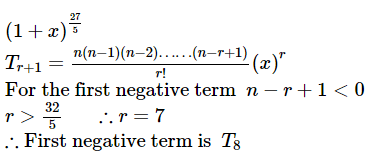QUESTION: 7

If w is a cube root of unity, then the value of (1 + ω - ω)2 (1 - ω + ω2) is

Solution:
QUESTION: 8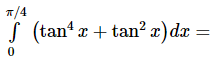Solution:
QUESTION: 9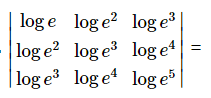Solution:
QUESTION: 10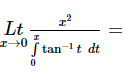Solution:
QUESTION: 11

The area bounded by the parabola y2=4ax and the straight line y=2ax is

Solution:

On solving y 2 = 4ax and y = 2ax, we get

x = 0 or 1 a
and y = 0 or 2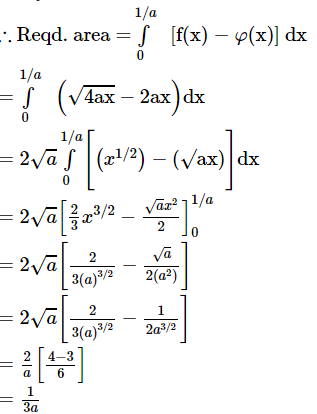QUESTION: 12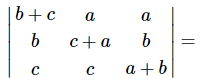Solution:
QUESTION: 13

If m and n are integers, then what is the value of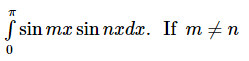Solution:

Since sin mx ,sin nx is an odd function if m ≠ n, then ∫ 0 π sin mx . sin nx dx = 0

QUESTION: 14

The particular integral of (D2 + 1)y = xe2x is equal to

Solution:
QUESTION: 15

The order and degree of the equation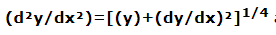are

Solution:
QUESTION: 16

If y=log logx, ey(dy/dx)=

Solution:
QUESTION: 17
(d/dx)(log tan x)=
Solution:
QUESTION: 18

If the normal at the point P(θ) to the ellipse ((x2/14)+(y2/5) = 1) intersects it again at the point Q(2θ), then cosθ is equal to

Solution:
QUESTION: 19
If the latus rectum of an ellipse is one half of its minor axis, then its eccentricity is
Solution:
QUESTION: 20

The parametric equations of the hyperbola x2/a2 - y2/b2 = 1 are

Solution:
QUESTION: 21

The eccentricity of the conic 9x2 - 16y2 = 144 is

Solution:
QUESTION: 22
Let f(x) = tan-1 {φ(x)}, where φ(x)is monotonically increasing for 0 < x < π/2. Then f(x) is
Solution: $f\prime \left(x\right)=\frac{\phi \prime \left(x\right)}{1+\left\{\phi \left(x\right){\right\}}^{2}}>\phantom{\rule{0.5em}{0ex}}\text{0 for 0}\phantom{\rule{0.5em}{0ex}}0,\phi \left(x\right)\phantom{\rule{0.5em}{0ex}}\text{being monotonically increasing}$
QUESTION: 23

Sin (sin⁻11/2 + cos⁻11/2) equals

Solution:
QUESTION: 24

If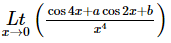is finite, then the values of a,b are respectively

Solution:
QUESTION: 25
If the value of a third order determinant is11, then the value of the square of the determinant formed by the co-factors will be
Solution:
QUESTION: 26

Largest value of min (2 + x2, 6 - 3x) when x > 0 is :

Solution:
QUESTION: 27
If a complex number lies in the IIIrd quadrant then its conjugate lies in quadrant number
Solution:
QUESTION: 28

The vertex of the parabola x 2 + 8 x + 12 y + 4 = 0 is

Solution:
QUESTION: 29

Axis of the parabola x 2 − 3 y − 6 x + 6 = 0 is

Solution:
QUESTION: 30
Out of 15 points in a plane, no three are in a straight line except 8 points which are collinear. How many triangles can be formed by joining them?
Solution:
QUESTION: 31
How many words can be formed from the letters of the word 'SIGNATURE' be arranged so that the vowels always come together?
Solution:
QUESTION: 32
In how many different ways can the letters of the word 'OPTICAL' be arranged so that the vowels always come together?
Solution:
QUESTION: 33

A box contains n enumerated articles. All the articles are taken out one by one at random. The probability that the numbers of the selected articles are in the sequence 1, 2, .........n is

Solution:
QUESTION: 34

An unbiased coin is tossed to get 2 points for turning up a head and one point for the tail. If three unbiased coins are tossed simultaneously, then the probability of getting a total of odd number of points is

Solution:
QUESTION: 35

In Δ A B C , i f b = 20 , c = 21 and sin A = 3 5 , then a =

Solution:
QUESTION: 36

Two dice are thrown simultaneously. The probability of getting a pair of 1 is

Solution:
QUESTION: 37

In a Δ A B C , 2s = perimeter and R = circumradius. Then s/R is equal to

Solution: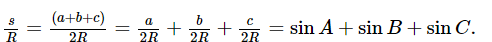QUESTION: 38

the greatest value of a non-negative real number λ for which both the equations 2x2 + (λ - 1)x + 8 = 0 and x2 - 8x + λ + 4 = 0 have real roots is :

Solution:
QUESTION: 39

The second drivative f"(x) of the function f(x) exists for all x in [0,1] and satisfies | f ″ x | ≤ 1. If f 0 = f 1 , then for all x in [0,1]

Solution:

The first derivative f'(x) exists for all x in [0,1] which implies that f(x) is continuous for all x in [0,1]. Also, it is given that f(0) = f(1)
Thus, applying Rolle's theorem on f(x) in the interval [0,1], we have
f'(c) = 0 for some c in [0,1]
The second derivative f''(x) exists for all x in [0,1] which implies that f'(x) is continuous for all x in [0,1]
Thus, applying Lagrange's theorem on f'(x) in the interval [ c , x ] c < x ≥ 1 , we have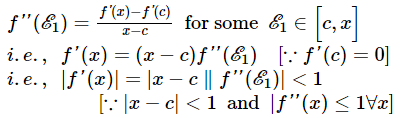Similarly, applying Lagrange's theorem on f’(x) in the interval [ x , c ] 0 ≤ x < c , we have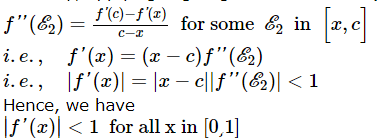QUESTION: 40

The set of values of p for which the roots of the equation 3x2 + 2x + (p - 1)p = 0 are of opposite sign, is

Solution:
QUESTION: 41

The fourth, seventh and tenth terms of a G.P. are p, q and r respectively, then

Solution:
QUESTION: 42

If A.M. between two numbers is 5 and their G.M. is 4, then their H.M. is

Solution:

If x, y and z respectively represent AM, GM and HM between two numbers a and b, then
y2 = xz
Here x = 5, y = 4
then 16 = 5x z
z = 16/5

QUESTION: 43
If 7th and 13th terms of an A.P. be 34 and 64 respectively, then its 18th term is
Solution:
QUESTION: 44
Every term of a G.P. is positive and also every term is the sum of two preceding terms. Then the common ratio of the G.P. is
Solution:
QUESTION: 45

If sin (x + 3α) = 3 sin (α - x), then

Solution:
QUESTION: 46

cot⁻1(-1/2) + cot⁻1(-1/3) is equal to

Solution:
QUESTION: 47
The coordinates of mid-point of portion of line cut by coordinate axis are (3,2), the equation of the line is
Solution:
QUESTION: 48

The angle between lines 3x+y-7=0 and x+2y+9=0 is

Solution:
QUESTION: 49
The condition that the cubic equation x3 - px2 + qx - r = 0 has all of its three roots equal is given by___
Solution: Let $\alpha$ is a root of the equation
${x}^{3}-p{x}^{2}+qx-r=0$
Since, all the roots of the given equation are equal
$\therefore \alpha +\alpha +\alpha =p$
$⇒\alpha =p∕3\phantom{\rule{0.5em}{0ex}}\phantom{\rule{0.5em}{0ex}}\phantom{\rule{0.5em}{0ex}}\dots \left(1\right)$
$\alpha .\alpha +\alpha .\alpha +\alpha .\alpha =q$
$⇒{\alpha }^{2}=q∕3\phantom{\rule{0.5em}{0ex}}\phantom{\rule{0.5em}{0ex}}\phantom{\rule{0.5em}{0ex}}\dots \left(2\right)$
$\alpha .\alpha .\alpha =r$
$⇒{\alpha }^{3}=r\phantom{\rule{0.5em}{0ex}}\phantom{\rule{0.5em}{0ex}}\phantom{\rule{0.5em}{0ex}}\phantom{\rule{0.5em}{0ex}}\dots \left(3\right)$
$\because {\left({\alpha }^{2}\right)}^{2}={\alpha }^{3}.\alpha$
$⇒{\left(q∕3\right)}^{2}=\left(r\right).\left(p∕3\right)$
$⇒{q}^{2}=3pr$
QUESTION: 50

I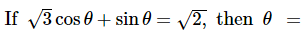Solution:
QUESTION: 51

The mean of the numbers a,b,8,5,10 is 6 and the variance is 6.80. Then which one of the following gives possible values of a and b?

Solution:
QUESTION: 52

A square piece of tin of side 18 cm is to be made into a box without top, by cutting a square from each corner and folding up the flaps to form the box. The maximum possible volume of the box is given by (in cm2)

Solution:
QUESTION: 53

The solution for the equation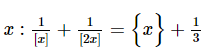Solution:
QUESTION: 54

On the interval [0, 1] the function
f x = x 1005 (1 − x )1002
assumes maximum value equal to

Solution:
QUESTION: 55

The value of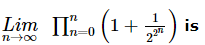Solution:
QUESTION: 56

Let a,b,c be any real numbers. Suppose that there are real numbers x,y,z not all zero such that x=cy+bz, y=az+cx and z=bx+ay. Then a2+b2+c2+2abc is equal to

Solution:
QUESTION: 57

is a binary operation defined on Q. Find which of the following operation is Associative.

Solution:
QUESTION: 58

In a triangle X Y Z , ∠ Z = π 2 . If tan X 2 and tan Y 2 are the roots of the equation ax2 + bx + c = 0, a ≠ 0 then

Solution:
QUESTION: 59

If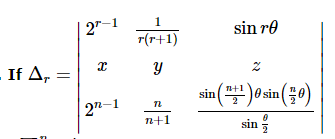then ∑ r = 1 n Δ r is equal to

Solution:
QUESTION: 60

Statement-1 :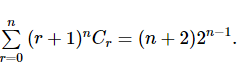Statement-2 :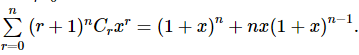Solution:
QUESTION: 61

Let P(3, 2, 6) be a point in space and Q be a point on the line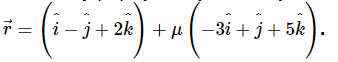Then the value of μ for which the vector P Q → is parallel to the plane x - 4y+3z = 1 is

Solution:
QUESTION: 62

Given the family of lines, a (3x + 4y +6) + b (x + y +2) = 0. The line of the family situated at the greatest distance from the point P(2, 3) has equation

Solution:
QUESTION: 63

The differential equation (x4 − 2 x y2 + y4 ) d x − 2x2 y − 4xy3 + sin y) dy = 0 has its solution as

Solution:
QUESTION: 64

If the function f(x) and g(x) are defined on RR such that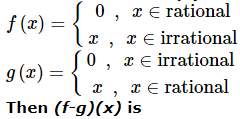Solution:
QUESTION: 65

The area bounded by the curves f (x)   = sin − 1 (sin x)    and (gx)   = [ sin − 1 sin x ] in the interval [ 0 , π ] , where [ . ] is a greatest integer function, is

Solution:
*Multiple options can be correct
QUESTION: 66

If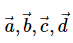are any four vectors then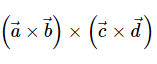is a vector

Solution:
*Multiple options can be correct
QUESTION: 67

The cubes of natural number are grouped as 1 3 , 2 3 , 3 3 , 4 3 , 5 3 , 6 3 ,...Let Sn denotes the sum of cubes in the nth group, then 8Sn is divisible by

Solution:
*Multiple options can be correct
QUESTION: 68

The solution of the equation cos 103 x − sin 103 x = 1 are

Solution:
*Multiple options can be correct
QUESTION: 69

In Δ A B C ,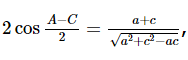c , then

Solution:
*Multiple options can be correct
QUESTION: 70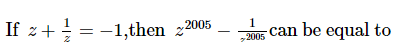Solution:
*Multiple options can be correct
QUESTION: 71

The point (0,0), (a, 11) and (b, 37) are the vertices of an equilateral triangle. Then

Solution:
*Multiple options can be correct
QUESTION: 72

If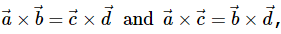then:

Solution:
*Multiple options can be correct
QUESTION: 73

For three vectors u → , v → , w → which of the following expression is not equal to any of the remaining three?

Solution:
*Multiple options can be correct
QUESTION: 74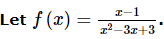. Then

Solution:
*Multiple options can be correct
QUESTION: 75

If a → = i + j ‸ + k ‸ , b → = 4 i ‸ + 3 j ‸ + 4 k ‸ and c → = i ‸ + α j ‸ + β k ‸ are linearly dependent vectors and | c | = 3 , then

Solution:Use Code STAYHOME200 and get INR 200 additional OFF Use Coupon Code